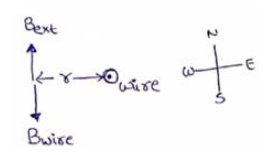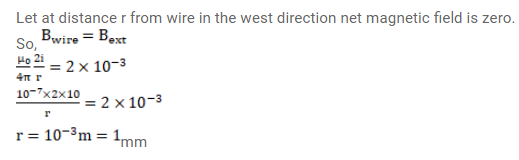# A long, vertical wire carrying a current of

Question:

A long, vertical wire carrying a current of $10 \mathrm{~A}$ in the upward direction is placed in a region where a horizontal magnetic field of magnitude $2.0 \times 10^{-3} \mathrm{~T}$ exists from south to north. Find the point where the resultant magnetic field is zero.

Solution: